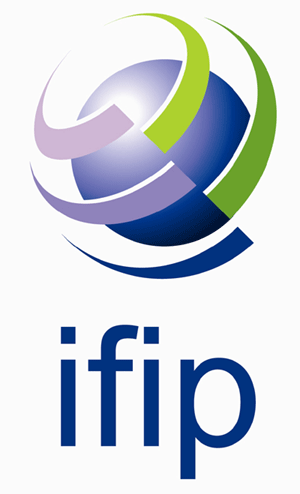27 th IFIP TC7 Conference 2015

on System Modelling and Optimization

SophiaTech Campus
Sophia Antipolis, France
June 29-July 3rd, 2015

Plenary Speakers

Plenary Speakers confirmed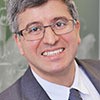"Bio-inspired imaging for medical applications" In this talk, we will suggest schemes for electrical sensing of objects by weakly electrical fish and for echolocation by bats. We will present recent results for shape identification and classification in electrosensing using pulse form and waveform signals. We will also introduce an efficient and novel approach based on frequency-dependent shape descriptors for shape classification in echolocation. Applications of the bio-inspired approaches in biomedical imaging for resolution, robustness, and specificity enhancement will be discussed."Control methods for the optimization of plasma scenarios in a Tokamak" A Tokamak is an experimental device, aiming to control nuclear fusion of light atoms by magnetic confinement. The plasma, which is made of electrons and ions, is governed, in the presence of a magnetic field, by the MHD (magnetohydrodynamics) equations. A typical discharge is made of a plasma current ramp-up, a plateau and a ramp-down phase. The goal of this work is to modelize a typical discharge and to compute the feedforward voltages to be applied to the external circuits in order to optimize, in a sense to be defined precisely, a scenario. Adjoint methods and optimization techniques will be used in order to perform this objective. Applications will be given for two Tokamaks: WEST (Tungsten Environment in steady-state Tokamak), which is an iron-transformer Tokamak, and ITER (International Thermonuclear Experimental Reactor), which is an air-transformer reactor, both located at Cadarache in the South of France."Stochastic derivative free algorithms for constrained optimization" In this talk we propose, analyse, and test algorithms for unconstrained and linearly constrained optimization when no use of derivatives of the objective function is possible. After a general introduction to the field, we will focus on model based algorithms in which the variable space is explored using samples of a sequence of probability distributions. We then show how to modify basic variant of the algorithm that yield a form of convergence to first order stationary points, even when the number of samples is limited. Two approaches are then described to handle constraints: the use of an extreme barrier function and the use of projection on the constraints. Numerical experiment on academic case and on a real-life industrial case illustrate to good performance and the reliability of our approach.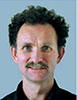Shape and Topology Optimization via the Level Set MethodShape and topology optimization via the level set method has started attracting the interest of an increasing number of researchers and industrial designers over the past years. A large number of academic problems, using various objective functions and constraints, have been successfully treated with this class of methods, showing its efficiency and flexibility. But real industrial applications may involve more complex and mixed constraints than classical optimal design problems. Moreover, they are sometimes not easy to formulate from a mathematical point of view, and even more difficult to handle numerically. Examples of such real life problems will be shown at the conference.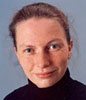Multiple Objective Optimization: Trading Off between Objectives, Constraints and Robustness Criteria In practical applications of Mathematical Optimization it is frequently observed that the decision maker prefers apparently suboptimal solutions. A natural explanation for this phenomenon is that the applied mathematical model did not fully represent all the decision makers criteria and constraints. Multiple objective optimization approaches are specifically designed to incorporate such complex preference structures, and they gain more and more importance in various application areas like engineering design and supply chain management. In this talk, we will link different concepts of constraint handling, robust optimization and stochastic programming, that usually have been considered fundamentally different, through the formulation of multiple objective counterparts. Assuming that the set of scenarios is finite, many uncertain optimization problems have a deterministic counterpart in this framework. Similarly, many different concepts of robust optimization and of stochastic programming can be described as special cases of a general nonlinear scalarization method applied to an associated multiple objective problem by choosing the involved parameters and sets appropriately. This forms the basis for an analysis of the interrelations and also the differences between established concepts in constrained optimization, robust optimization and stochastic programming. By providing additional trade-off information between alternative efficient solutions, the multiple objective counterpart can facilitate the decision making process when deciding for a most preferred solution.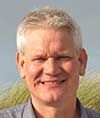"Fourier Methods and Second Order Weak Taylor Scheme for Backward Stochastic Differential Equations in Computational Finance"In this presentation we will explain how we can solve linear, semi-linear as well as nonlinear partial differential equations by the concept of backward stochastic differential equations, Fourier cosine expansions and wavelets. We will discuss the highly efficient pricing of financial options in the Fourier context. We extend the Fourier method to solve so-called decoupled forward-backward stochastic differential equations (FBSDEs) with second-order accuracy. The FSDE is approximated by different Taylor schemes, such as the Euler, Milstein, and Order-2.0-weak-Taylor schemes. The conditional expectations appearing are approximated by using the characteristic function for these schemes.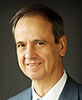"On the modeling of clouds in the context of variational inequalities" After some brief remarks on the mathematical and numerical modelling of the atmosphere and the oceans, we describe some new developments related to the modelling of the humid atmosphere with saturation. Because of the change of phase, this problem leads to discontinuous nonlinear equations, which are not of classical type: issues of modeling, existence of solutions, uniqueness, computation which appear in this context will be discussed.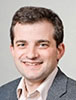"Sparse Control Problems in Measure Spaces" In this talk we consider optimal control problem governed by elliptic and parabolic equations, where the control variable lies in a measure space. Such formulations lead to a sparse structure of the optimal control, which provides among other things an elegant way to attack problems of optimal source placement as well as point source identification problems. We discuss the functional analytic settings of such problems and the regularity issues of the optimal solutions. Moreover, we present a discretization concept and provide a priori and a posteriori error estimates for the discretization error. Numerical examples for elliptic and parabolic problems in two and three space dimensions illustrate our results.